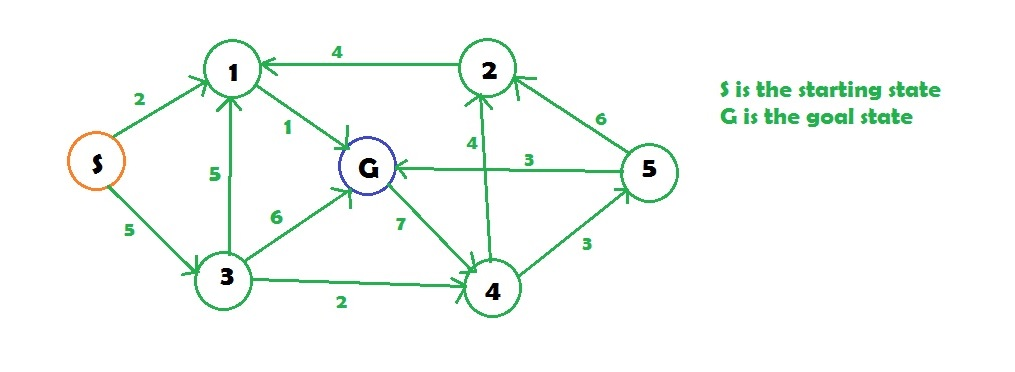# Uniform-Cost Search (Dijkstra for large Graphs)

Uniform-Cost Search is a variant of Dijikstra’s algorithm. Here, instead of inserting all vertices into a priority queue, we insert only source, then one by one insert when needed. In every step, we check if the item is already in priority queue (using visited array). If yes, we perform decrease key, else we insert it.
This variant of Dijsktra is useful for infinite graphs and those graph which are too large to represent in the memory. Uniform-Cost Search is mainly used in Artificial Intelligence.

Examples:

```Input :Output :
Minimum cost from S to G is =3
```

## Recommended: Please try your approach on {IDE} first, before moving on to the solution.

Uniform-Cost Search is similar to Dijikstra’s algorithm . In this algorithm from the starting state we will visit the adjacent states and will choose the least costly state then we will choose the next least costly state from the all un-visited and adjacent states of the visited states, in this way we will try to reach the goal state (note we wont continue the path through a goal state ), even if we reach the goal state we will continue searching for other possible paths( if there are multiple goals) . We will keep a priority queue which will give the least costliest next state from all the adjacent states of visited states .

 `// C++ implemenatation of above approach ` `#include ` `using` `namespace` `std; ` ` `  `// graph ` `vector > graph; ` ` `  `// map to store cost of edges ` `map, ``int``> cost; ` ` `  `// returns the minimum cost in a vector( if  ` `// there are multiple goal states) ` `vector<``int``> uniform_cost_search(vector<``int``> goal, ``int` `start) ` `{ ` `    ``// minimum cost upto ` `    ``// goal state from starting ` `    ``// state ` `    ``vector<``int``> answer; ` ` `  `    ``// create a priority queue ` `    ``priority_queue > queue; ` ` `  `    ``// set the answer vector to max value ` `    ``for` `(``int` `i = 0; i < goal.size(); i++) ` `        ``answer.push_back(INT_MAX); ` ` `  `    ``// insert the starting index ` `    ``queue.push(make_pair(0, start)); ` ` `  `    ``// map to store visited node ` `    ``map<``int``, ``int``> visited; ` ` `  `    ``// count ` `    ``int` `count = 0; ` ` `  `    ``// while the queue is not empty ` `    ``while` `(queue.size() > 0) { ` ` `  `        ``// get the top element of the  ` `        ``// priority queue ` `        ``pair<``int``, ``int``> p = queue.top(); ` ` `  `        ``// pop the element ` `        ``queue.pop(); ` ` `  `        ``// get the original value ` `        ``p.first *= -1; ` ` `  `        ``// check if the element is part of ` `        ``// the goal list ` `        ``if` `(find(goal.begin(), goal.end(), p.second) != goal.end()) { ` ` `  `            ``// get the position ` `            ``int` `index = find(goal.begin(), goal.end(),  ` `                             ``p.second) - goal.begin(); ` ` `  `            ``// if a new goal is reached ` `            ``if` `(answer[index] == INT_MAX) ` `                ``count++; ` ` `  `            ``// if the cost is less ` `            ``if` `(answer[index] > p.first) ` `                ``answer[index] = p.first; ` ` `  `            ``// pop the element ` `            ``queue.pop(); ` ` `  `            ``// if all goals are reached ` `            ``if` `(count == goal.size()) ` `                ``return` `answer; ` `        ``} ` ` `  `        ``// check for the non visited nodes ` `        ``// which are adjacent to present node ` `        ``if` `(visited[p.second] == 0) ` `            ``for` `(``int` `i = 0; i < graph[p.second].size(); i++) { ` ` `  `                ``// value is multiplied by -1 so that  ` `                ``// least priority is at the top ` `                ``queue.push(make_pair((p.first +  ` `                  ``cost[make_pair(p.second, graph[p.second][i])]) * -1,  ` `                  ``graph[p.second][i])); ` `            ``} ` ` `  `        ``// mark as visited ` `        ``visited[p.second] = 1; ` `    ``} ` ` `  `    ``return` `answer; ` `} ` ` `  `// main function ` `int` `main() ` `{ ` `    ``// create the graph ` `    ``graph.resize(7); ` ` `  `    ``// add edge ` `    ``graph.push_back(1); ` `    ``graph.push_back(3); ` `    ``graph.push_back(1); ` `    ``graph.push_back(6); ` `    ``graph.push_back(4); ` `    ``graph.push_back(6); ` `    ``graph.push_back(2); ` `    ``graph.push_back(5); ` `    ``graph.push_back(1); ` `    ``graph.push_back(2); ` `    ``graph.push_back(6); ` `    ``graph.push_back(4); ` ` `  `    ``// add the cost ` `    ``cost[make_pair(0, 1)] = 2; ` `    ``cost[make_pair(0, 3)] = 5; ` `    ``cost[make_pair(1, 6)] = 1; ` `    ``cost[make_pair(3, 1)] = 5; ` `    ``cost[make_pair(3, 6)] = 6; ` `    ``cost[make_pair(3, 4)] = 2; ` `    ``cost[make_pair(2, 1)] = 4; ` `    ``cost[make_pair(4, 2)] = 4; ` `    ``cost[make_pair(4, 5)] = 3; ` `    ``cost[make_pair(5, 2)] = 6; ` `    ``cost[make_pair(5, 6)] = 3; ` `    ``cost[make_pair(6, 4)] = 7; ` ` `  `    ``// goal state ` `    ``vector<``int``> goal; ` ` `  `    ``// set the goal ` `    ``// there can be multiple goal states ` `    ``goal.push_back(6); ` ` `  `    ``// get the answer ` `    ``vector<``int``> answer = uniform_cost_search(goal, 0); ` ` `  `    ``// print the answer ` `    ``cout << ``"Minimum cost from 0 to 6 is = "`  `         ``<< answer << endl; ` ` `  `    ``return` `0; ` `} `

Output:

```Minimum cost from 0 to 6 is = 3
```

Complexity: O( m ^ (1+floor(l/e)))
where,
m is the maximum number of neighbor a node has
l is the length of the shortest path to the goal state
e is the least cost of an edge

My Personal Notes arrow_drop_upCheck out this Author's contributed articles.

If you like GeeksforGeeks and would like to contribute, you can also write an article using contribute.geeksforgeeks.org or mail your article to contribute@geeksforgeeks.org. See your article appearing on the GeeksforGeeks main page and help other Geeks.

Please Improve this article if you find anything incorrect by clicking on the "Improve Article" button below.